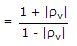# Electronics and Communication Engineering - Microwave Communication

### Exercise :: Microwave Communication - Section 2

11.

If a line having Z0 = 300 ∠ 0 W is open circuited at far end, VSWR is

 A. 0 B. 1 C. ∞ D. 2

Answer: Option C

Explanation:

If line is o.c. |rv| = 1 and VSWR= ∞.

12.

If f is the frequency of electromagnetic wave, fc is cutoff frequency, then in a rectangular waveguide

 A. attenuation is low when f > fc B. attenuation is low when f < fc C. attenuation is high when f < fc D. either (b) or (c)

Answer: Option A

Explanation:

Wavelength should be less than cutoff wavelengths.

Therefore frequency should be higher than cutoff frequency for minimum attenuation.

13.

Assertion (A): The condition of minimum distortion in a transmission line is L = RC/G.

Reason (R): Line loading means addition of inductance to satisfy the condition L = RC/G.

 A. Both A and R are correct and R is correct explanation of A B. Both A and R are correct but R is not correct explanation of A C. A is correct but R is wrong D. A is wrong but R is correct

Answer: Option B

Explanation:

Oscillator circuits using vacuum tubes (triodes, pentodes) have the following limitations at very high frequencies (microwave region).

The stray capacitances and inductances become important and affect the operation of the circuit.

At low frequencies the transit time between cathode and anode is a small fraction of period of oscillation.

However, at microwave frequencies this transit time becomes comparable to time period of oscillations.

14.

A magnetron has a cylindrical cathode surrounded by an anode structure having cavities opening into interaction space by means of slots

 A. True B. False

Answer: Option A

Explanation:

It is somewhat similar to TWT and can deliver microwave power over a wide frequency band.

It has an electron gun and a helix structure. However the interaction between electron beam and RF wave is different than in TWT.

The growing RF wave travels in opposite direction to the electron beam.

The frequency of wave can be changed by changing the voltage which controls the beam velocity.

Moreover the amplitude of oscillations can be decreased continuously to zero by changing the beam current.

It features are:

1. Frequency range - 1 GHz to 1000 GHz.

2. Power output - 10 mV to 150 mW (continuous wave) 250kW (pulsed).

It is used as signal source in transmitters and instruments.

15.

The correct sequence of parts in klystron amplifier are

 A. anode, catcher cavity, cathode, buncher cavity B. cathode, buncher cavity, catcher cavity, cavity C. anode, buncher cavity, catcher cavity, cathode D. cathode, catcher cavity, anode, buncher cavity

Answer: Option B

Explanation:

Cathode is the first part and anode is the last.

#### Current Affairs 2021

Interview Questions and Answers